# DAX In Power BI - Learn Power BI DAX Basics

Are you aware of why DAX in Power BI is critical for data analytics? Your decision-making is influenced by the establishment of bespoke metrics. Well, you may have already imported some data into Power BI desktop files and even created reports that provide useful information. DAX functions in Power BI and how to create measurements using the Power BI desktop will be covered in this article.

Rating: 5

7777

Do you know why the DAX in Power BI plays a vital role in data analytics? How does the creation of custom measures impact your decision-making?

Well, You might’ve imported some data into Power BI desktop files and even create reports that show valuable insights. But what if, you need to analyze the growth (%) of product lists over different date ranges or, need to analyze the annual growth of your company compared to rivals? This is where Power BI DAX comes into the picture.

In this blog, we’re going to discuss the most useful DAX functions in Power BI and the creation of measures by using the Power BI desktop and more.

## DAX In Power BI

Power BI DAX Tutorial | DAX Basics in Power BI | DAX Functions in Power BISo, let us begin with the DAX definition first, which I am going to talk about in the section below.

## DAX Basics in Power BI: What is DAX?

Data Analysis Expressions (DAX) is a syntax language that comprises formulae and expressions that are used in data manipulation. Functions, constants, and operators are used in DAX to create expressions. In simpler terms, DAX is the advanced version of MS Excel with high-end data manipulation and management capabilities. It is developed by Microsoft to interact with its business intelligence and data modeling tools like PowerPivot, Power BI. Power BI is a robust analytics tool by Microsoft that offers several features and functions using DAX as a language.

Some of the major advantages of Power BI are:

• Real-time data visualization
• Creation of charts and report generation on voice command using Cortana
• Large storage space and easy retrieval of data from the storage
• Higher security at the Row level
• BI has intuitive user experience features and memorizes the most frequently used trends

All these functions are essentially performed by pre-set tools and commands in the system. But there are several occasions when you want to perform a particular type of function says a certain type of data analysis but you are unable to do so because the command does not pre-exist in the system. What do you do in such a case? This is where DAX comes into the picture.

 Want to be part of the Power BI real-time environment? then visit here Microsoft Power BI Certification Course

### Importance of DAX In Power BI

DAX is quite an important syntax as working in DAX improves the user experience by deploying functionalities like data visualization, data importing, and manipulating. For normal report creation, the basic knowledge of the dashboard is enough but to be able to build up sophisticated and insightful reports, DAX is required. With several commands and measures that can be used under the DAX syntax, the reports generated are highly intuitive and discerning.

### DAX in Power BI: How does it work?

DAX works on a combination of three fundamental concepts:

1. Syntax
2. Context
3. Functions

These inputs are fundamental to the creation of a specific command that fetches desired results.

#### 1. Syntax

Syntax refers to the components of the formula you are making. It is the language used in the formula like the command, sign, operators, destination column or row or table, etc. For example, name, parenthesis, summation, name of the table, etc.

#### 2. Context

Context refers to the target row that has been included in the formula for data retrieval or calculation. Context is present in two types: Row Context and Filter Context.

#### 3. Functions

Functions refer to the predefined or already existing commands in the system. For example, Sum, Add, True, False, etc.

 Also Read:  Power BI Architecture Tutorial

### DAX in Power BI: Calculated Columns & Measures

In this article, we are going to be focusing on the Power BI DAX formulae used in calculations, Measures, and Calculated Columns.

#### #1. Calculated Column

Similar to other regular columns, calculated columns are the ones that come out as a result of computations within two columns of different data sets. They are ideal for row-wise calculations;

#### #2. Calculated Measure

Calculated columns are ideal for dynamic calculations as they do not acquire any physical space. These are used when data in rows are grouped together for computations.

#### Calculated Columns vs Measures

Calculated columns and calculated measures both use DAX expressions for data manipulation. The difference lies in the context of the underlying formula. In the calculated column type, the calculation takes place at the row level within a given table. In the calculated measure type, the calculation is done at the cell level in the entire report or the query.

### Power BI DAX Basics: Types of Functions in DAX

#### #1. Aggregate Functions

MIN

Fetches the minimum value in a given column.

Syntax

``MIN(<column>)``

Example

``=MIN( [ SellerMargin] )``

MINA

Fetches the minimum value along with Aggregate Functions Logical values and text representation of numbers if any

Syntax

``MINA( <column> )``

Example

``=MINA( InternetSales[Freight] )``

MINX

Fetches the minimum value after evaluation of each row expression in a given table.

Syntax

``MINX  ( < table >, <expression> )``

Example

`` =MINX(  FILTER( InternetSales ) ,  [ SalesTerittoryKey ] = 2 ,  [Freight] )``

Other functions

• MAX
• MAXA
• MAXX
• SUM
• AVERAGE
• SUMX
• AVERAGEX
 Checkout Power Bi Desktop Tutorial

#### #2. Count Functions

DISTINCTCOUNT

Fetches the count of distinct numbers avoiding any replication.

Syntax

``DISTINCTCOUNT( <column> )``

Example

``=DISTINCTCOUNT( ProductsList[ProductID] )   ``

COUNT

Fetches the total count of items even if repetitions are present.

Syntax

`` COUNT( <column> )``

Example

``=COUNT ( [ShipDate]  )   ``

COUNTA

Fetches the count of items in a non-empty column.

Syntax

``COUNTA( <column> )``

Example

``= COUNTA( ‘ProductSeller’[Phone] )   ``

COUNTROWS

Fetches the number of rows in a given table.

Syntax

``COUNTROWS( < table > )``

Example

``=COUNTROWS( ‘Enquiries’ )``
 Visit here to know the difference between Tableau Vs Power BI

#### #3. Date-Time Functions

DATE

Fetches the desired date in Date-time format.

Syntax

``DATE ( <year>, <month>, <day> )``

Example

``=DATE ( 2020,02,27 )  ``

HOUR

Fetches hours in the AM PM format.

Syntax

``HOUR ( <datetime> )``

Example

``=HOUR( ‘Orders’ [TransactionTime] )``

TODAY

Fetches the current date.

Syntax

``TODAY()``

Example

`` = YEAR ( TODAY())-2012``

Other functions

• NOW
• EOMONTH
 Visit here to know the Power BI Slicers - A Complete Guide

#### #4. Math Functions

ABS

Fetches the absolute value.

Syntax

``ABS( <number> )``

Example

``=ABS( [LabelPrice] - [SellingPrice] )   ``

EXP

Fetches the exponent's value.

Syntax

``EXP( <number> )``

Example

``=EXP( [Power] )  ``

FACT

Fetches the factorial of a given number.

Syntax

``FACT( <number> )``

Example

``=FACT( [Values] )``

Other functions

• LN
• LOG
• PI
• POWER
• QUOTIENT
• SIGN
• SQRT

#### #5. Logical Functions

AND

Performs the logical conjunction on two specified expressions.

Syntax

``AND( <logical1> , <logical2 > )``

Example

`` =IF( AND(1<2 , 2<3) , “All true” , “One or false” ) ``

OR

Performs the logical disjunction on two specified expressions.

Syntax

``OR( <logical1 > , <logical2 > )``

Example

``=( IF(OR(1<2 , 2<3) , “All true” , “One or more false” )   ``

NOT

Performs logical negation on the given expression.

Syntax

``NOT( <logical > )``

Example

`` =NOT( [ProductPrices] )``

Other functions

• IF
• IFERROR

#### #6. Information Functions

ISBLANK

Declares if the value is blank or not as true or false.

Syntax

``ISBLANK( <value> )``

Example

``=IF( ISBLANK(‘CalculatedMeasures’[PreviousYearTotalSales]) ,BLANK() , (CalculatedMeasures’[PreviousYearTotalSales]/ ‘CalculatedMeasures’[PreviousyearTotalSales])``

ISNUMBER

Declares if the value is a number or not.

Syntax

``ISNUMBER(<value>)``

Example

``=IF( ISNUMBER(2), “Is number”, “Is Not number” )   ``

ISNONTEXT

Declare if the value is non-text.

Syntax

`` ISNONTEXT( <value> )``

Example

``=IF( ISNONTEXT(“ ”), “Is Non-Text”, “Is Text” )``

Other functions

• ISERROR
• ISTEXT

#### #7. Text Functions

CONCATENATE

Performs joining of two strings.

Syntax

``CONCATENATE( <text1> , <text2> )``

Example

``= CONCATENATE( “Hello” , “Learner” )     ``

FIXED

Performs rounding off of number to a given decimal.

Syntax

``FIXED( <number> , <decimals> , <no_commas> )``

Example

``=FIXED( [LabelPrice], 3,1 )   ``

REPLACE

Replaces part of a string with specified characters.

Syntax

``REPLACE( <old_text> , <start_num>, <num_chars> , <new_text> )``

Example

``=REPLACE( ‘New Services’[Service ID],2,3,”AB” )``

Other functions:

• SEARCH
• CONCATENATEX
• SEARCH
• UPPER
 Also Read: Power BI Services and Benefits

### Creating a Measure using Power BI DAX

The following steps make your task simple to create a measure using Power BI DAX.

1. First, go to the Power BI Desktop app.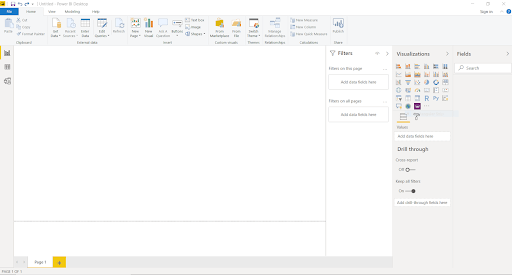2. Right-click the Sales table or hover over More options (...) of Field pane.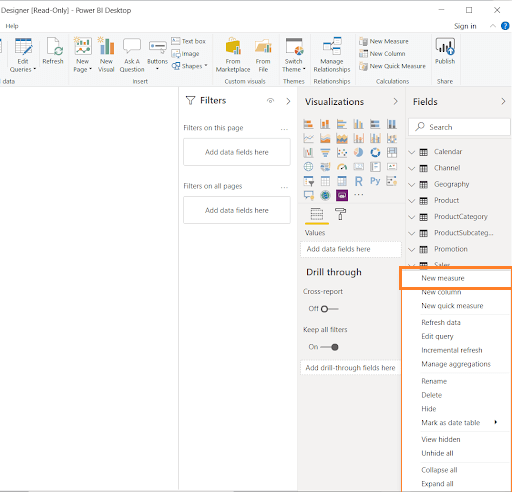3. Once the menu is presented, select  New Measure.  This activity stores the new Measure in the Sales table. You can also create a new Measure from the Power BI desktop ribbon in the following way.

Go to   Home tab > Calculations group > New Measure.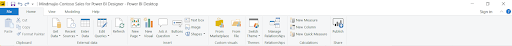4. Next, the Formula bar exists on top of the reporting canvas, where you can edit your name and write a  Data Analysis Xpression formula.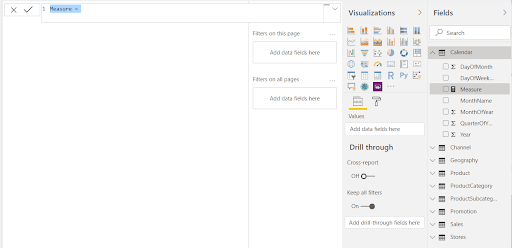Let’s discuss the concept before creating the formula. This makes it simple to proceed further

Variables Selection and Formula creation:

• The net amount of any products or services requires some variables.so, I listed out prerequisite variables:  Net Amount, Quantity of products or services, price of each quantity, and other expenditure(Total cost price).
• Net amount = (Total Quantity Sales) * (Per/Product or Service) - Expenditure (cost price).

5. The Measure is named by default in the formula bar, but we need Measure to be more noticeable, emphasize Measure in the formula bar and alter it to  Net Amount.

6. While creating your formula, write Sum after the equal sign. Now, the drop-down suggestion list appears, once you type the starting of the letter. Select from the list, if needed, and then press Enter.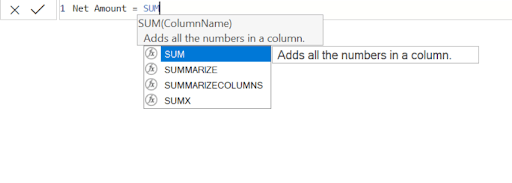7. Press enter after typing the SUM. Now, you can see an open parenthesis along with a drop-down suggestion list of existing columns. It can be passed to the SUM function.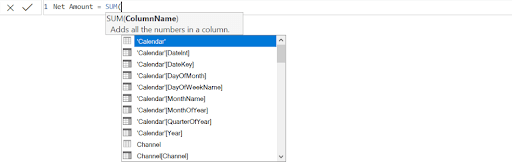8. The expression is always between Open parenthesis and Closing parenthesis. For this instance, your expression comprises an argument to push to the SUM function: the SalesQuantity Column. Start Typing SalesQuantity until Sales[SalesQuantity] is the only value that appears in the list.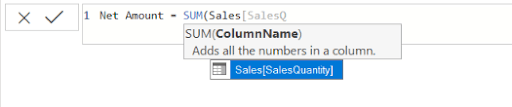The Table name is preceded by the column name is called the fully qualified name of the column. It makes your formula easier to comprehend.

9. Select Sales[Sales quantity] from the list then enter closing parenthesis.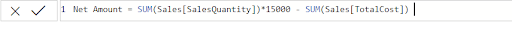Multiply the Value with the First Expression and Subtract the other Column from the Final Value:

• After finishing the first expression, multiply 15,000 with that expression to obtain the total amount of quantity.

Sales[SalesQuantity]* 15000.

• Type a space after the desired value, space, a minus operator (-), and then another space.
• Write another SUM function, and Start writing TotalCost you can select the Sales[TotalCost]  and then enter closing parenthesis.

10. To finish and validate the formula, we need to enter either Enter or Select CheckMark icon(Commit).

The validated Net Amount measure is now ready to use in the sales table in the Fields pane.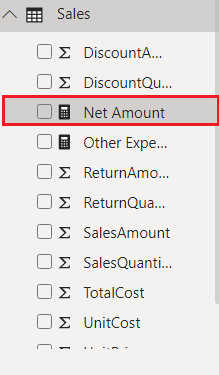### Conclusion:

DAX is one of the most commonly used expression syntax these days with a wide range of applicability. Learning it opens up several opportunities in the market, especially in SQL-enabled companies. With DAX in Power BI, you are empowered to build up any kind of logical expression or formula as per requirement.

Reach out to us in the comments if you want to learn and excel in DAX.Course Schedule
NameDates
Power BI TrainingMay 30 to Jun 14
Power BI TrainingJun 03 to Jun 18
Power BI TrainingJun 06 to Jun 21
Power BI TrainingJun 10 to Jun 25
Last updated: 29 May 2023